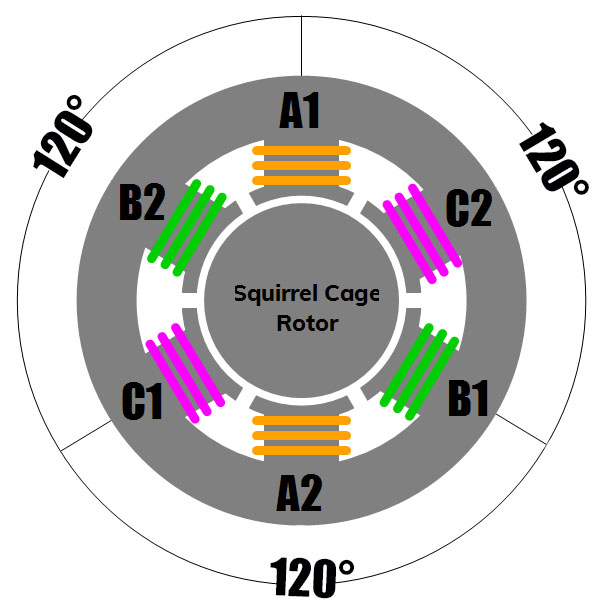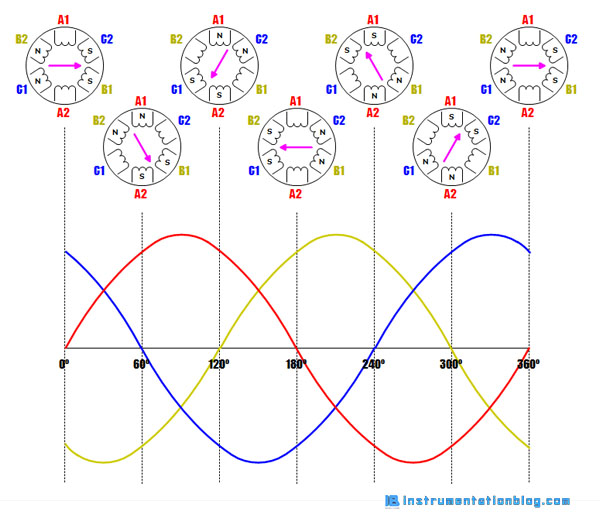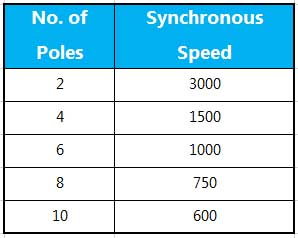# How rotating magnetic field works in an Induction Motor?

## How does a rotating magnetic field work?

When a three-phase supply is given to an ac induction motor, it will generate a rotating magnetic field that rotates at a synchronous speed.

In this article, we are going to see about the basic theory of rotating magnetic field. For that, we have to first understand electromagnetism.

### What is Electromagnetism?

Electromagnetism is a process to generate a magnetic field by electrically charging a conductor. When a current flows through a conductor, it generates a magnetic field around the conductor. The size of a magnetic field completely depends on the amount of current flow.A relationship between the direction of current flow and the direction of a magnetic field is shown from the below image. If a current-carrying conductor is grasped with the left hand with the thumb pointing in the direction of electron flow, the fingers will point in the direction of the magnetic lines of flux.An electromagnet can be made by winding a piece of a conductor through the coil and then applying a voltage to that coil. A current through the coil generates a magnetic field. The strength of the magnetic field can be increased by increasing the number of turns in the coil.

The magnetic field of an electromagnet has the same characteristics as the natural magnet including the north and south poles. When the direction of the current flow changes, it will change the polarity of the electromagnet.

### How does a rotating magnetic field works in an induction motor?

As we have seen the construction of AC motor in my previous article. We have seen that the stator of an ac motor consists of stator windings and a three-phase supply is applied to the stator windings.The following image shows that the six coils(two coils for each phase) are used in the stator windings. These coils operate in a pair, each coil operates as an electromagnet. As we have seen from the above image that the coils are wound in such a way that when a current flows through them, one coil becomes a north pole and the other coil becomes a south pole.

For example, a three-phase supply is applied to the stator windings. Phase A is connected to the phase-A of the motor, the same way phase-B and phase-C are connected to the phase-B and phase-C of the motor.

Each phase has a phase angle of 120º apart. Consider an example for a two-pole stator, here each phase windings appear two times. The magnetic field developed in phase windings depends on the direction of current flow through that winding.To understand the rotating magnetic field, let’s take an example of a two-pole stator in which we will see the one complete rotation of the rotating magnetic field.We have seen phase-A, phase-B and phase-C indicated as red, yellow, and blue color. Each phase is 120º apart from the other phase. Each phase consists of two coils. as phase-A has A1 and A2 coils, respectively phase-B has B1 and B2 and phase-C has C1 and C2 coils.

At 0º ⇒ Phase-A is natural, no current flows through it.

Phase-B has current flow in a negative direction, that’s why B1 becomes south and B2 becomes north. Phase-C has current flow in a positive direction, that’s why C1 becomes north and C2 becomes south.

Magnetic lines of flux leave from B2 and C1 and enters into the C2 and B1 poles. The arrow indicates the magnetic field.

At 60º ⇒ Phase-C becomes neutral, Phase-A has current flow in a positive direction, that’s why A1 becomes north and A2 becomes south. Phase-B has current flow in a negative direction, that’s why B1 becomes south and B2 becomes north.

Magnetic lines of flux leave from A1 and B2 and enters into the B1 and A2 poles. The magnetic field is rotated by 60º.

At 120º ⇒ Phase-B becomes neutral, Phase-A has current flow in a positive direction, that’s why A1 becomes north and A2 becomes south. Phase-C has current flow in a negative direction, that’s why C1 becomes south and C2 becomes north.

Magnetic lines of flux leave from A1 and C2 and enters into the A2 and C1 poles. The magnetic field is rotated again by 60º.

At the end of 360°, the magnetic field will have rotated one full revolution of 360°. This process will repeat 50 times a second on a 50 Hz power supply.

### What is Synchronous Speed?

The speed of the rotating magnetic field is referred to as synchronous speed. Synchronous speed is equal to 120 times the frequency, divided by the number of poles.

Below is the simple formula to find out the synchronous speed,

Ns = (120F/P), where F=frequency and N=Number of Poles

As an example, the synchronous speed for a two-pole motor operated at a frequency of 50Hz is 3000 rpm.

The synchronous speed decreases as the number of poles increases.The most used 3 Basic Motor Starter with its PLC Program!

What is Temperature Scanner? How does it work?

What is the Servo Motor? How does it work?

How star-delta starter works?

What is Soft Starter? How it works?

Circuit Breaker Fundamentals.

Relay Working and its importance in an electrical field.

You can read more articles about Electrical and you can also find books that boost your knowledge in the field of Instrumentation

1.